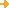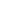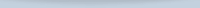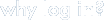search:advanced search
(24 resources)

Resources

 This article from the magazine The Economist argues that the notion of mathematical proof is now in flux and that "the use of computers to prove mathematical theorems is forcing mathematicians to re-examine the... https://www.economist.com/science-and-technology/2005/03/31/... QuickMath is one of the most useful sites for common but tedious mathematical calculations. It is a free "online calculator that solves equations and does all sorts of algebra and calculus problems." Separated into many... https://quickmath.com/ A discussion of a variety of strategies for math problem solving, with examples of how to use them and why the development of problem-solving skills is important. The unit is accompanied by a kit of materials available... https://teachersinstitute.yale.edu/curriculum/guides/1980/7/... Teaching word problems and methods of solution: a unit concerned with practical approaches to problem solving at the elementary mathematical level. It presents concepts and ideas calculated to encourage educators to... https://teachersinstitute.yale.edu/curriculum/guides/1980/7/... A unit that introduces flowcharting as a technique for solving math word problems in a logical, ordered sequence. Basic charting concepts of categorizing data and information in groups of input and output data,... https://teachersinstitute.yale.edu/curriculum/guides/1981/6/... An introductory review of basic skills and functions, focusing on playing games with numbers, learning logical thinking, and working on exercises that emphasize developing alternate ways of thinking, important precursors... https://teachersinstitute.yale.edu/curriculum/guides/1980/4/... Simple constructions; constructing regular polyhedra; symmetry; and some group theory. https://teachersinstitute.yale.edu/curriculum/guides/1987/5/... The Electronic Journal of Differential Equations (EJDE) is a collection of research articles "related to differential equations and their applications." There are over 50 articles for the current volume, possibly with... https://ejde.math.txstate.edu/ Maths Challenge is a fun activity to build mathematical and reasoning skills. Each month during the school year, a new set of math problems are posted online. There are miscellaneous problems at junior and senior levels,... https://mathschallenge.net/ Several online mathematical utilities intended for college and university students (math, physics, engineering, etc. students). Numerical utilities to solve (among others): N Equations in N Unknowns, Eigenvalues and... http://www.akiti.ca/Mathfxns.html

Switch to browsing by Resource Type
Switch to browsing by Format
Switch to browsing by Education Level
Switch to browsing by LCC (More Detailed Classifications)
Switch to browsing by Key Conceptforget your password?Save, organize, and share resources that you find.

Subscribe to bulletins

It's easy, fast, and FREE!get your own loginAMSER =
FREE ONLINE
RESOURCES
for the
CLASSROOM